The K5 Learning Blog urges parents to be pro-active in helping their children reach their full academic potential.

K5 Learning
provides an online reading and math program for kindergarten to grade 5 students.Learn More about K5's online learning program.

# How do you Measure the Area of Parallelograms, Triangles and Trapezoids?

In grade 5, students learn to measure the areas of parallelograms, triangles without right angles and trapezoids. What are these shapes and how do you calculate the area of each? In this post, we’ll go through each shape and show you how to calculate the areas.

## What is a parallelogram?

A parallelogram is a flat shape with opposite sides parallel and equal in length. This is what it looks like: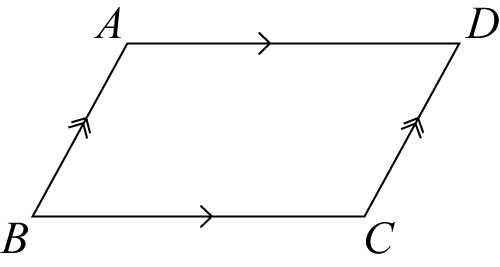The opposite sides are parallel.
The opposite sides are equal in length.
The opposite angles are equal. In our diagram, that is, the angle of A and C are the same, and the angle of B and D are the same.

Note: squares, rectangles and rhombuses are all parallelograms.

## How do you calculate the area of a parallelogram?

The area of a parallelogram is the base times the height.

Area = b x h

For example: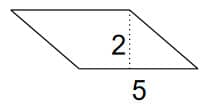This parallelogram has a base of 5 and a height of 2. What is the area?

b = 5
h = 2

A = b x h; A = 5 x 2 = 10. The area is 10.

## What is a triangle?

We all know what a triangle looks like: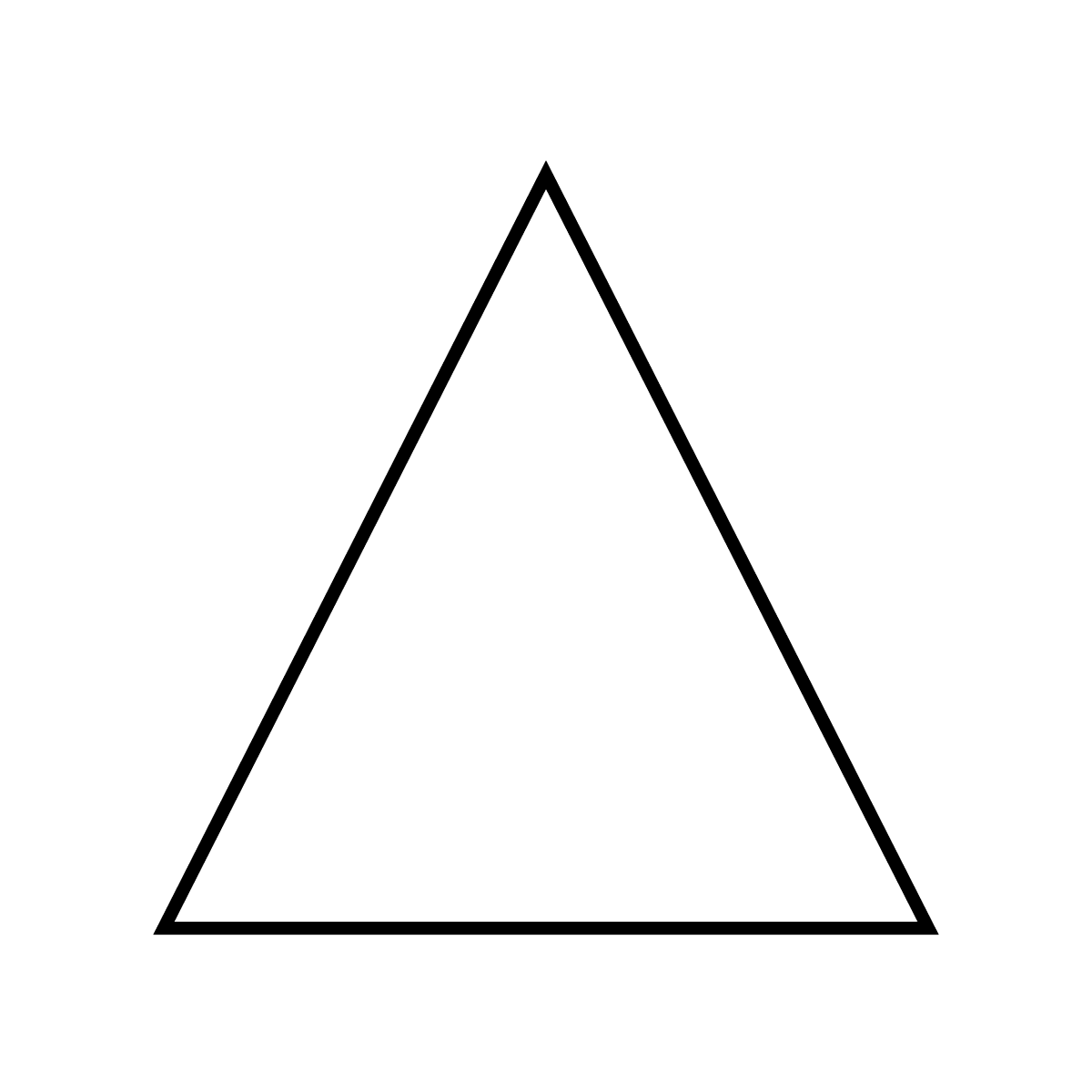What does a triangle without right angles look like? It would look something like this: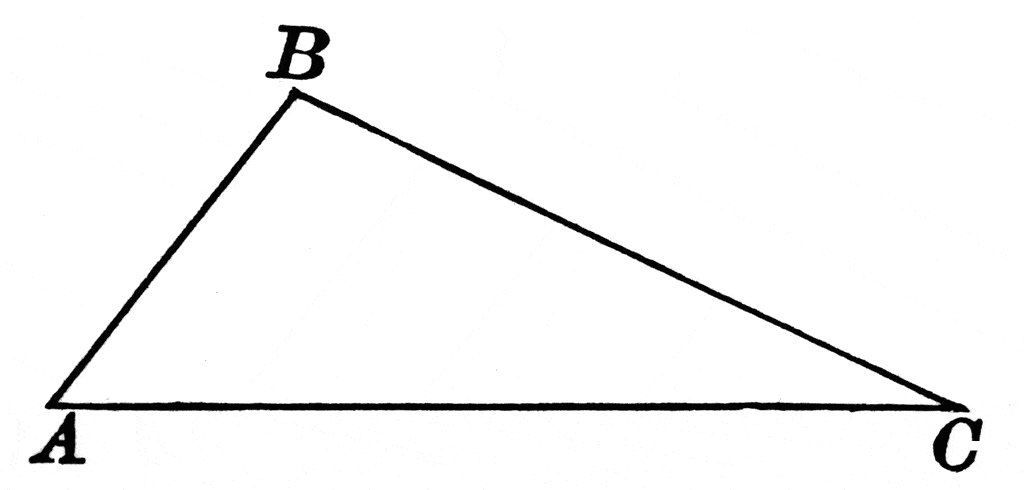## How do you calculate the area of a triangle without right angles?

The area of a triangle is half of base times height.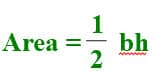Let’s look at this in an example: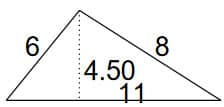b = 11
h = 4.5

Area = ½ 11 x 4.5 = 24.75. The area is 24.75.

## What is a trapezoid?

A trapezoid is a 4-sided flat shape with straight sides that has a pair of opposite sides that are parallel. It looks like this: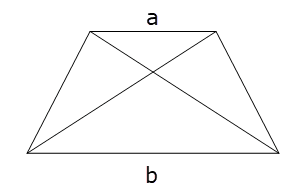## How do you calculate the area of a trapezoid?

The area of a trapezoid is the average of the two base lengths (a + b) times the height.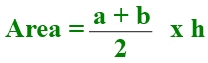Here’s an example: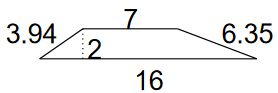The answer is: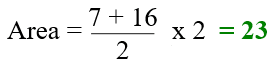The area of the trapezoid is 23.

If you are looking for practice in calculating the areas of these shapes, you can find worksheets on this grade 5 geometry page:

Area of triangles, trapezoids and parallelograms